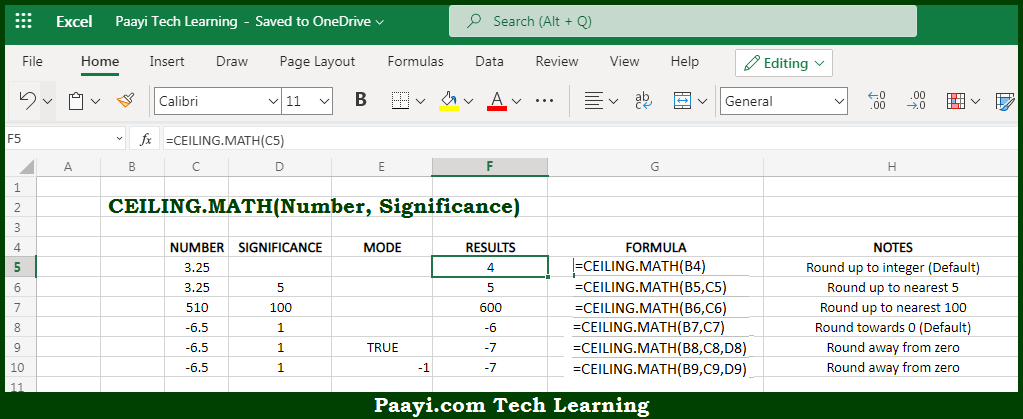# Learn How to Use Microsoft Excel CEILING.MATH Function

Written by | 0 Comments | 614 Views

In this article, you will learn how to use Microsoft Excel CEILING.MATH function and its prime function in Microsoft Excel. You will also get to know the Microsoft Excel CEILING.MATH function returns value and syntax with the help of some examples.

Microsoft Excel CEILING.MATH Function

The main function of Microsoft Excel CEILING.MATH function is to round up a number to the nearest multiple. So, with the help of CEILING.MATH function, you can able to round up the number to the nearest specified multiple. The function CEILING.MATH function operates differently from CEILING function, as CEILING.MATH defaults to a multiple of 1, and offers absolute control over the rounding direction for negative numbers. So, with the help of Microsoft Excel CEILING.MATH function you can easily round up the number to the nearest multiple.

Return Value of CEILING.MATH Function

The return value will be the rounded number.

Syntax of CEILING.MATH Function

=CEILING.MATH(number, [significance], mode)

Where the arguments:

• number: This is the number you want to round up.
• significance: This is the multiple used while rounding up the number, default is 1 (optional).
• mode: It is used for rounding negative numbers towards or away from 0, default is 0 (optional).

## How to Use Microsoft Excel CEILING.MATH Function?Microsoft Excel CEILING.MATH function is used to round up a number up to a nearest integer or multiple of significance. By default, CEILING.MATH rounds to the nearest integer, using a significance of 1. Positive numbers with decimal portions are rounded up to the nearest integer and negative numbers with decimal portions are rounded toward zero. Mode defaults to zero.

When the mode is zero or removed, CEILING.MATH rounds negative numbers toward zero. When a mode is of any non-zero value, CEILING.MATH rounds negative numbers away from zero. The mode does not have any effect on positive numbers. So, with the help of Microsoft Excel CEILING.MATH function you can easily round up the number to the nearest multiple.